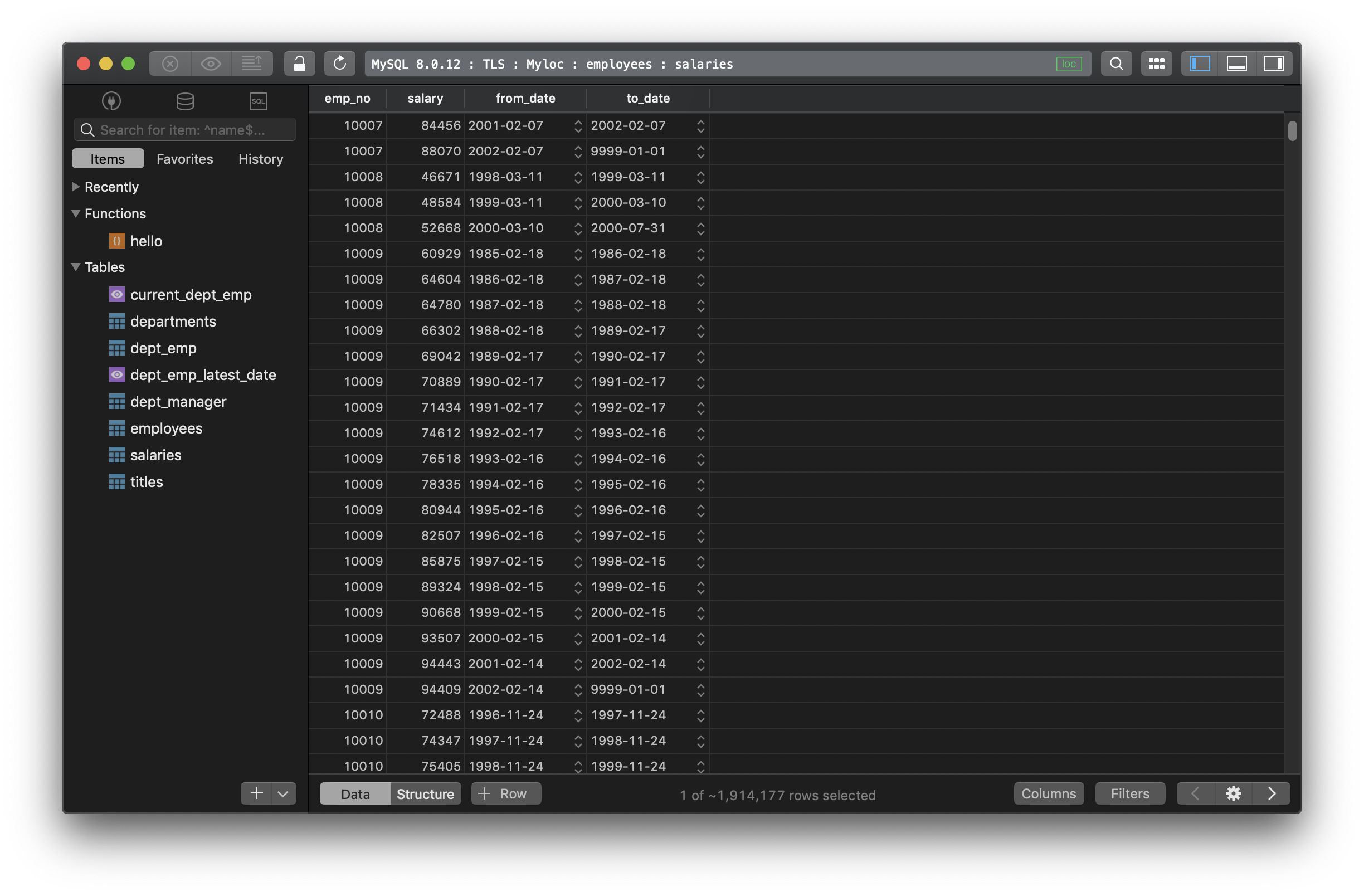In SQL, there are 4 arithmetic operators to perform arithmetical operations on numeric operands involved: addition(`+`), subtraction(`-`), multiplication(`*`) and division(`/`)

Addition(`+`) and subtraction(`-`) operations can also be used in date arithmetic.

Here is the generic syntax for using the arithmetic operators:

``````expression arithmetic_operator expression
``````

For subtraction:

``````expression - expression
``````

The expression can be of any numeric data types, except bit value.

For example, use subtraction in `SELECT` query:

``````SELECT MAX(salary) - MIN(salary) AS salary_range
FROM employees;
``````

Need a good GUI tool for databases? TablePlus provides a native client that allows you to access and manage Oracle, MySQL, SQL Server, PostgreSQL, and many other databases simultaneously using an intuitive and powerful graphical interface.